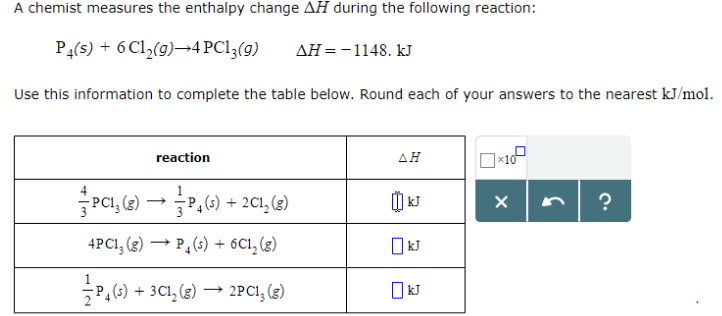# Problem: A chemist measures the enthalpy change ΔH during the following reaction: P4 (s) + 6Cl2 (g) → 4 PCl3 (g)       ΔH = -1148.kJ Use this information to complete the table below. Round each of your answers to the nearest kJ/mol.

###### FREE Expert Solution
99% (199 ratings)###### Problem Details

A chemist measures the enthalpy change ΔH during the following reaction:

P4 (s) + 6Cl2 (g) → 4 PCl3 (g)       ΔH = -1148.kJ

Use this information to complete the table below. Round each of your answers to the nearest kJ/mol.